# Biorhythms

Biorhythms were invented over 100 years ago and entered our popular culture in the 1960s. You can still find many Web sites today that offer to prepare personalized biorhythms, or that sell software to compute them. Biorhythms are based on the notion that three sinusoidal cycles influence our lives. The physical cycle has a period of 23 days, the emotional cycle has a period of 28 days, and the intellectual cycle has a period of 33 days. For any individual, the cycles are initialized at birth. All the people on earth born on one particular day share the biorhythm determined by that date.

### Contents

#### Personal biorhythm

I hope you can download the latest version of my biorhythm program from MATLAB Central at this link. If you already happen to have access to the programs from my book "Experiments with MATLAB", you will find an earlier version of biorhythm there. Either of these programs will allow you to compute your own personal biorhythm.

#### Vectorized plots

Biorhythms are an excellent way to illustrate MATLAB's vector plotting capabilities. Let's start with a column vector of time measured in days.

t = (0:28)';


The first way you might think of to compute the biorhythm for these days is create this array with three columns.

y = [sin(2*pi*t/23) sin(2*pi*t/28) sin(2*pi*t/33)];


Or, we can get fancy by moving the square brackets inside the sin(...) evaluation and, while we're at it, define an anonymous function. This vectorized calculation is the core of our biorhythm function.

bio = @(t) sin(2*pi*[t/23 t/28 t/33]);
y = bio(t);


We're now ready for our first plot. Because t goes from 0 to 28, the 23-day blue curve covers more than its period, the 28-day green curve covers exactly one period, and the 33-day red curve covers less its period.

clf
plot(t,y)
axis tight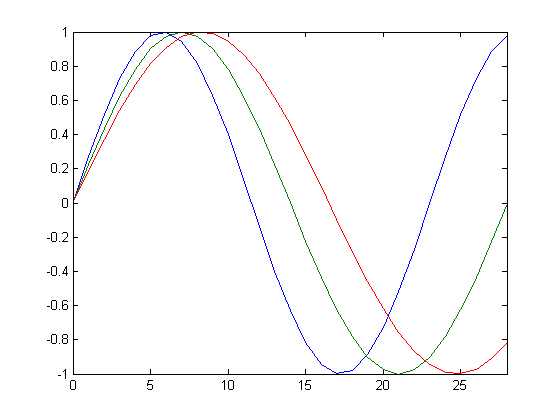#### Date functions

MATLAB has several functions for computations involving calendars and dates. They are all based on a date number, which is the amount of time, in units of days, since an arbitrary origin in year zero. The current datenum is provided by the function now. I am writing this post on June 10, 2012, and every time I publish it, the value of now changes. Here is the current value.

format compact
format bank
date = now

date =
735030.87


A readable form of the current date is provided by datestr.

datestr(now)

ans =
10-Jun-2012 20:45:47


The integer part of now is a date number for the entire day. This value is used in biorhythm.

format short
today = fix(now)

today =
735030


#### Newborn

With no input arguments, my latest version of biorhythm plots the biorhythm for a baby born four weeks before today. The plot covers the eight week period from the baby's birth until a date four weeks in the future. You can see the three curves all initialized at birth. The blue physical cycle has a 23-day period, so it passes through zero five days before today. The green emotional cycle has a 28-day period, so it hits zero today. And, the red intellectual cycle will be zero five days from today.

biorhythm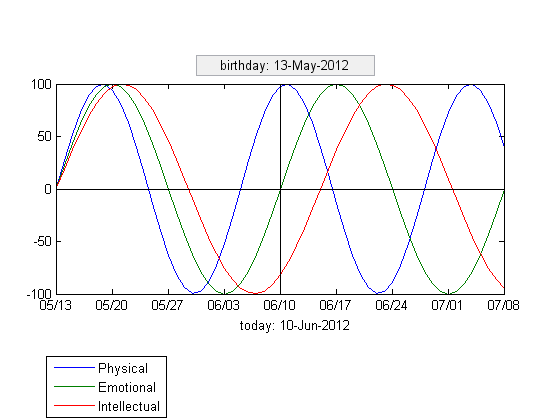#### Twenty-first Birthday

Let's look at the biorhythm for someone whose 21st birthday is today. The date vector for such a birthday is obtained by subtracting 21 from the first component of today's datevec. This is a pretty unexciting, but typical, biorhythm. All three cycles are near their midpoints. Blue and red peaked about a week ago and green will peak a little more than a week from now.

birthday = datevec(today) - [21 0 0 0 0 0];
biorhythm(birthday)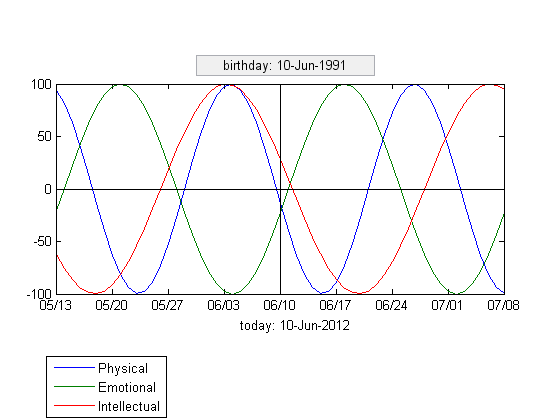#### Rebirth

Does your biorhythm ever start over? Yes, it does, at a time t when t/23, t/28 and t/33 are all integers. Since the three periods are relatively prime, the first such value of t is their product.

tzero = 23*28*33

tzero =
21252


How many years and days is this?

dpy = 365+97/400         % 365 days/year + 97/400 for leap years.
yzero = fix(tzero/dpy)
dzero = dpy*mod(tzero/dpy,1)

dpy =
365.2425
yzero =
58
dzero =
67.9350


So, your biorhythm starts over when you are 58 years old, about 68 days after your birthday.

biorhythm(today-tzero)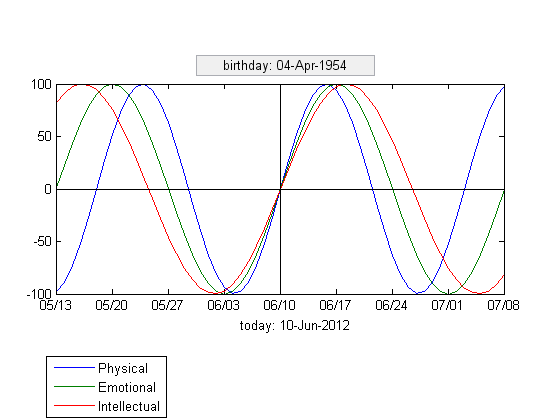#### Nearly Perfect Day

Is there ever a perfect day, one where all three cycles reach their maximum at the same time? Well, not quite. That would require a time t when, for p = 23, 28, 33, t/p = n+1/4, where n is an integer. Then sin(2*pi*t/p) would equal sin(pi/2), which is the maximum. But, since the values of p are relatively prime, there is no such value of t. But we can get close. To find the nearly perfect day, look for the maximum value of the sum of the three cycles.

t = (1:tzero)';
y = bio(t);
s = sum(y,2);
top = find(s==max(s))
biorhythm(today-top)

top =
17003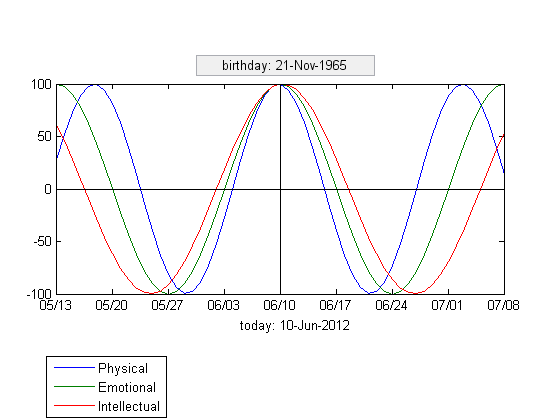How old are you on this nearly perfect day?

top/dpy

ans =
46.5526


So, half-way through your 46th year.

#### Detail

But the nearly perfect day is not perfection. The three cyles are not quite at their peaks.

bio(top)

ans =
0.9977    1.0000    0.9989


Let's zoom in to a two day window around top, the location of the maximum sum. Measure time in hours.

clf
t = (-1:1/24:1)';
y = 100*bio(top+t);
plot(24*t,y)
set(gca,'xaxislocation','top','xlim',[-24 24],'xtick',-24:6:24,...
'ylim',[96.0 100.4])
title(['biorhythms near day ' int2str(top) ', time in hours'])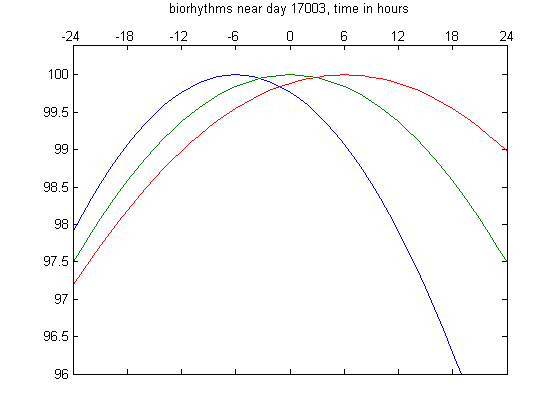We can see that the three peaks occur six hours apart. This is the closest we get to perfection, and the only time in the entire 58-year cycle when we get even this close.

Have a good day.

Published with MATLAB® 7.14

|